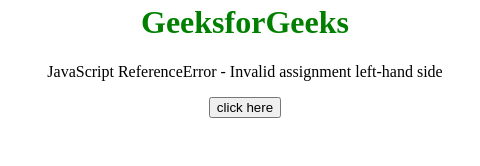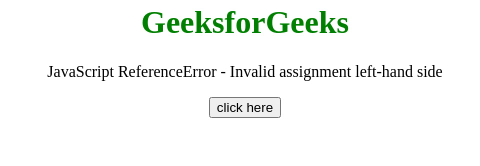# JavaScript ReferenceError – Invalid assignment left-hand side

Basic Example of ReferenceError – Invalid assignment left-hand side, run the code and check the console

Example:

## Javascript

 ``

Output:

`ReferenceError: Invalid left-hand side in assignment`

This JavaScript exception invalid assignment left-hand side occurs if there is a wrong assignment somewhere in code. A single “=” sign instead of “==” or “===” is an Invalid assignment.

Message:

```ReferenceError: invalid assignment left-hand side
```

Error Type:

```ReferenceError
```

Cause of the error: There may be a misunderstanding between the assignment operator and a comparison operator.

Example 1: In this example, “=” operator is misused as “==”, So the error occurred.

## HTML

 ` ` `<``html``> ` `    ``<``head``> ` `    ``<``body` `style``=``"text-align: center;"``> ` `        ``<``h1` `style``=``"color: green;"``> ` `            ``GeeksforGeeks ` `        `` ` `        ``<``p``> ` `            ``JavaScript ReferenceError -  ` `            ``Invalid assignment left-hand side ` `        `` ` ` `  `        ``<``button` `onclick``=``"Geeks();"``> ` `            ``click here ` `        `` ` `        ``<``p` `id``=``"GFG_DOWN"``> ` ` `  `        ``<``script``> ` `            ``var el_down = document.getElementById("GFG_DOWN"); ` `            ``function Geeks() { ` `                ``try { ` `                    ``if ((Math.PI = 10 || Math.PI = 5)) { ` `                        ``document.write("Inside Loop"); ` `                    ``} ` `                    ``el_down.innerHTML =  ` `                      ``"'Invalid assignment left-hand side'" +  ` `                      ``" error has not occurred"; ` `                ``} catch (e) { ` `                    ``el_down.innerHTML =  ` `                      ``"'Invalid assignment left-hand side'" +  ` `                      ``"error has occurred"; ` `                ``} ` `            ``} ` `        `` ` `    `` ` ``

Output:Example 2: In this example, the + operator is used with the declaration, So the error has not occurred.

## HTML

 ` ` `<``html``>   ` `<``head``>      ` `    ` `<``body` `style``=``"text-align:center;"``>  ` `    ``<``h1` `style``=``"color:green;"``>   ` `        ``GeeksforGeeks   ` `    ``  ` `    ``<``p``> ` `        ``JavaScript ReferenceError - ` `        ``Invalid assignment left-hand side ` `    `` ` `    `  `    ``<``button` `onclick` `= ``"Geeks();"``> ` `        ``click here ` `    `` ` `    ``<``p` `id``=``"GFG_DOWN"``>  ` `    `` ` `        `  `    ``<``script``>  ` `        ``var el_down = document.getElementById("GFG_DOWN"); ` `        ``function Geeks() {  ` `            ``try { ` `                ``var str = 'Hello, ' ` `                ``+ 'Geeks'; // Error Here ` `                ``el_down.innerHTML =  ` `                ``"'Invalid assignment left-hand side'"+ ` `                ``"error has not occurred"; ` `            ``} catch(e) { ` `                ``el_down.innerHTML =  ` `                ``"'Invalid assignment left-hand side'"+ ` `                ``"error has occurred"; ` `            ``} ` `        ``}  ` `    ``  ` `    ` ``

Output:My Personal Notes arrow_drop_upCheck out this Author's contributed articles.

If you like GeeksforGeeks and would like to contribute, you can also write an article using contribute.geeksforgeeks.org or mail your article to contribute@geeksforgeeks.org. See your article appearing on the GeeksforGeeks main page and help other Geeks.

Please Improve this article if you find anything incorrect by clicking on the "Improve Article" button below.

Article Tags :

Be the First to upvote.

Please write to us at contribute@geeksforgeeks.org to report any issue with the above content.